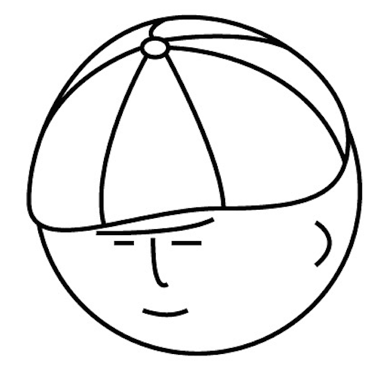# マツシタのお勉強メモ

#### マツシタのお勉強

##### welcome to my engineering blog
###### Hacker Rank# Problem

https://leetcode.com/problems/longest-substring-with-at-most-k-distinct-characters/

Given a string, find the length of the longest substring T that contains at most `k` distinct characters.

For example, Given s = "eceba" and k = 2,

T is "ece" which its length is 3.

# How to Solve

This problem can be solved by InchWorm Method(しゃくとり法). Solution is the following steps.

1. I get `right` go on till restriction that count of distinct characters has to be below number `K` is broken
2. in case of breaking above restriction, I calculate length of substring.
3. I get `left` go on till that restriction is met.
4. I repeat from step1 to step3 till right reaches size of string which is input value
5. In step 4, I return the max value in all size of subtring